×#### Thank you for registering.

One of our academic counsellors will contact you within 1 working day.

Click to Chat

1800-1023-196

+91-120-4616500

CART 0

• 0

MY CART (5)

Use Coupon: CART20 and get 20% off on all online Study Material

ITEM
DETAILS
MRP
DISCOUNT
FINAL PRICE
Total Price: Rs.

There are no items in this cart.
Continue Shopping• Complete JEE Main/Advanced Course and Test Series
• OFFERED PRICE: Rs. 15,900
• View Details

```Chapter 3: Pair of Linear Equations in Two Variables Exercise – 3.8

Question: 1

The numerator of a fraction is 4 less than the denominator. If the numerator is decreased by 2 and denominator is increased by 1, then the denominator is eight times the numerator. Find the fraction.

Solution:

Let the numerator and denominator of the fraction be x and y respectively. Then the fraction is x/y

The numerator of the fraction is 4 less the denominator. Thus, we have

x = y – 4

⇒ x – y = − 4

If the numerator is decreased by 2 and denominator is increased by 1, then the denominator is 8 times the numerator. Thus, we have

y + 1 = 8(x - 2)

⇒ y + 1 = 8x – 16

⇒ 8x – y = 1 + 16

⇒ 8x – y = 17

So, we have two equations

x – y = - 4

8x – y = 17

Here x and y are unknowns. We have to solve the above equations for x and y. Subtracting the second equation from the first equation, we get

(x – y) – (8x – y) = – 4 – 17

⇒ x − y − 8x + y = −21

⇒ −7x = −21

⇒ −7x = −21

⇒ x = 21/7

⇒ x = 3

Substituting the value of x in the first equation, we have

3 – y = – 4

⇒ y = 3 + 4

⇒ y = 7

Hence the fraction is 3/7

Question: 2

A fraction becomes 9/11 if 2 is added to both numerator and the denominator. If 3 is added to both the numerator and the denominator it becomes 5/6. Find the fraction

Solution:

Let the numerator and denominator of the fraction be x and y respectively. Then the fraction is x/y

If 2 is added to both numerator and the denominator, the fraction becomes 9/11. Thus, we have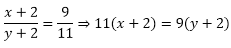⇒ 11x + 22 = 9y + 18 ⇒ 11x – 9y = 18 – 22 ⇒ 11x – 9y + 4 = 0

If 3 is added to both numerator and the denominator, the fraction becomes 5/6⇒ 6(x + 3) = 5(y + 3)

⇒ 6x + 18 = 5y + 15

⇒ 6x – 5y = 15 –18

⇒ 6x – 5y + 3 = 0

So, we have two equations

11x – 9y + 4 = 0

6x – 5y + 3 = 0

Here x and y are unknowns. We have to solve the above equations for x and y.

By using cross-multiplication, we havex = 7, y = 9

The fraction is 7/9

Question: 3

A fraction becomes 1/3 if 1 is subtracted from both its numerator and denominator. If 1 is added to both the numerator and denominator, it becomes 1/2. Find the fraction.

Solution:

Let the numerator and denominator of the fraction be x and y respectively. Then the fraction is x/y. If 1 is subtracted from both numerator and the denominator, the fraction becomes 1/3. Thus, we have⇒3(x – 1) = (y – 1)

⇒ 3x – 3 = y – 1

⇒ 3x – y – 2 = 0

If 1 is added to both numerator and the denominator, the fraction becomes 1/2. Thus, we have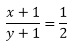⇒ (2x + 1) = (y + 1) ⇒ 2x + 2 = y + 1

⇒ 2x – y + 1 = 0

So, we have two equations

3x – y – 2 = 0

2x – y + 1 = 0

Here x and y are unknowns. We have to solve the above equations for x and y.

By using cross-multiplication, we have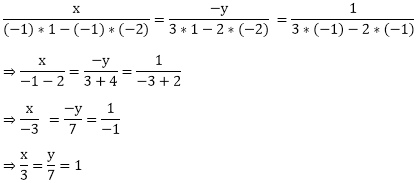⇒ x = 3, y = 7

The fraction is 3/7

Question: 4

If we add 1 to the numerator and subtract 1 from the denominator, a fraction becomes 1. It also becomes 1/2 if we only add 1 to the denominator. What is the fraction?

Solution:

Let the numerator and denominator of the fraction be x and y respectively. Then the fraction is x/y.

If 1 is added to the numerator and 1 is subtracted from the denominator, the fraction becomes 1. Thus, we have⇒(x + 1) = (y–1)

⇒ x + 1– y + 1 = 0

⇒ x – y + 2 = 0

If 1 is added to the denominator, the fraction becomes 1/2. Thus, we have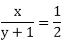⇒ 2x = (y + 1)

⇒ 2x – y – 1 = 0

So, we have two equations

x – y + 2 = 0

2x – y – 1 = 0

Here x and y are unknowns. We have to solve the above equations for x and y.

By using cross-multiplication, we have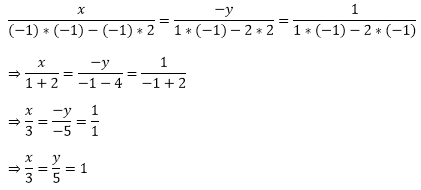⇒ x = 3, y = 5

The fraction is 3/5

Question: 5

The sum of the numerator and denominator of a fraction is 12. If the denominator is increased by 3, the fraction becomes 1/2. Find the fraction.

Solution:

Let the numerator and denominator of the fraction be x and y respectively. Then the fraction is x/y

The sum of the numerator and denominator of the fraction is 12. Thus, we have

x + y = 12

⇒ x + y – 12 = 0

If the denominator is increased by 3, the fraction becomes 1/2. Thus, we have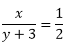⇒ 2x = (y + 3)

⇒ 2x – y – 3 = 0

So, we have two equations

x + y – 12 = 0

2x – y – 3 = 0

Here x and y are unknowns. We have to solve the above equations for x and y.

By using cross-multiplication, we have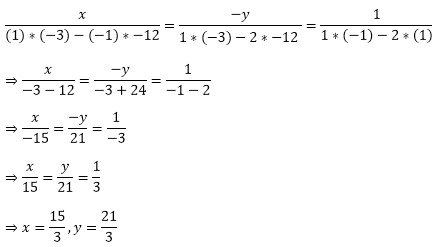⇒ x = 5, y = 7

The fraction is 5/7

Question: 6

The sum of a numerator and denominator of a fraction is 18. If the denominator is increased by 2, the fraction reduces to 1/3. Find the fraction.

Solution:

Let the numerator and denominator of the fraction be x and y respectively. Then the fraction is x/y

The sum of the numerator and denominator of the fraction is 18. Thus, we have

x + y = 18

⇒ x + y – 18 = 0

If the denominator is increased by 2, the fraction becomes 1/3. Thus, we have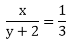⇒ 3x = (y + 2)

⇒ 3x – y – 2 = 0

⇒ 3x – y – 2 = 0

So, we have two equations

x + y – 18 = 0

3x – y – 2 = 0

Here x and y are unknowns. We have to solve the above equations for x and y.

By using cross-multiplication, we have⇒ x = 5, y = 13

The fraction is 5/13

Question: 7

If 2 is added to the numerator of a fraction, it reduces to 1/2 and if 1 is subtracted from the denominator, it reduces to 1/3. Find the fraction.

Solution:

Let the numerator and denominator of the fraction be x and y respectively. Then the fraction is x/y.

If 2 is added to the numerator of the fraction, it reduces to 1/2. Thus we have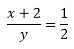⇒ 2(x + 2) = y

⇒ 2x + 4 = y

⇒ 2x – y + 4 = 0

If 1 is subtracted from the denominator, the fraction reduces to 1/3. Thus, we have⇒ 3x = (y – 1)

⇒ 3x –y + 1 = 0

So, we have two equations

2x – y + 4 = 0

3x – y + 1 = 0

Here x and y are unknowns. We have to solve the above equations for x and y.

By using cross-multiplication, we have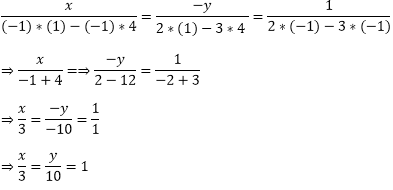⇒ x = 3, y = 10

The fraction is 3/10

Question: 8

The sum of the numerator and denominator of a fraction is 4 more than twice the numerator. If the numerator and denominator are increased by 3, they are in the ratio 2: 3. Determine the fraction.

Solution:

Let the numerator and denominator of the fraction be x and y respectively. Then the fraction is x/y.

The sum of the numerator and denominator of the fraction is 4 more than twice the numerator. Thus, we have

x + y = 2x + 4

⇒ 2x + 4 – x – y = 0

⇒ x – y + 4 = 0

If the numerator and denominator are increased by 3, they are in the ratio 2 : 3. Thus we have

x + 3: y + 3 = 2: 3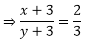⇒3(x + 3) = 2(y + 3)

3x + 9 = 2y + 6

⇒ 3x – 2y + 3 = 0

So, we have two equations

x – y + 4 = 0

3x – 2y + 3 = 0

Here x and y are unknowns. We have to solve the above equations for x and y.

By using cross-multiplication, we have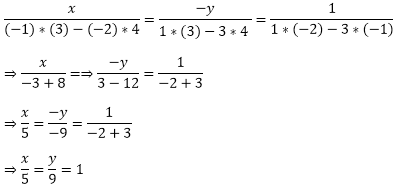⇒ x = 5, y = 9

The fraction is 5/9

Question: 9

If the numerator of a fraction is multiplied by 2 and the denominator is reduced by 5 the fraction becomes 6/5. And, if the denominator is doubled and the numerator is increased by 8, the fraction becomes 2/5. Find the fraction.

Solution:

Let the numerator and denominator of the fraction be x and y respectively. Then the fraction is x/y. If the numerator is multiplied by 2 and denominator is reduced by 5, the fraction becomes 6/5. Thus, we have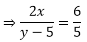⇒ 10x = 6(y – 5)

⇒ 10x – 6y + 30 = 0

⇒ 2(5x - 3y + 15) = 0

⇒ 5x - 3y + 15 = 0

If the denominator is doubled and the numerator are increased by 8, the fraction becomes 2/5. Thus we have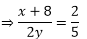⇒ 5(x + 8) = 4y

⇒ 5x + 40 = 4y

5x – 4y + 40 = 0

So, we have two equations

5x – 3y + 15 = 0

5x – 4y + 40 = 0

Here x and y are unknowns. We have to solve the above equations for x and y.

By using cross-multiplication, we have⇒ x = 12, y = 25

The fraction is 12/25

Question: 10

The sum of the numerator and denominator of a fraction is 3 less than twice the denominator. If the numerator and denominator are decreased by 1, the numerator becomes half the denominator. Determine the fraction

Solution:

Let the numerator and denominator of the fraction be x and y respectively. Then the fraction is x/y.

The sum of the numerator and denominator of a fraction is 3 less than twice the denominator. Thus, we have

x + y = 2y – 3

⇒ x + y – 2y + 3 = 0

⇒ x – y + 3 = 0

If the numerator and denominator are decreased by 1, the numerator becomes half the denominator. Thus, we have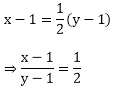⇒2(x - 1) = (y – 1)

⇒ 2x – 2 = (y – 1)

⇒ 2x – y – 1 = 0

So, we have two equations

x – y + 3 = 0

2x – y – 1 = 0

Here x and y are unknowns. We have to solve the above equations for x and y.

By using cross-multiplication, we have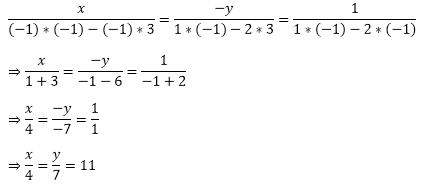⇒ x = 4, y = 7

The fraction is 4/7
```### Course Features

• 728 Video Lectures
• Revision Notes
• Previous Year Papers
• Mind Map
• Study Planner
• NCERT Solutions
• Discussion Forum
• Test paper with Video Solution